首页   注册   登录
V2EX = way to explore
V2EX 是一个关于分享和探索的地方

Meteor
JSLint - a JavaScript code quality tool
jsFiddle
D3.js
WebStorm

JavaScript 权威指南第 5 版Closure: The Definitive GuideV2EX  ›  JavaScript

# JavaScript 函数式编程初窥

vivaxy · 47 天前 · 721 次点击

## 编程范式

• 命令式编程
• 面向对象编程
• 函数式编程

### 不同的编程范式有不同的代码表现``````const app = 'github';
const greeting = 'Hi, this is ';
console.log(greeting + app);
``````

``````const Program = function() {
this.app = 'github';
this.greeting = function() {
console.log('Hi, this is ' + this.app);
};
};
const program = new Program();
program.greeting();
``````

``````const greet = function(app) {
return 'Hi, this is ' + app;
};
console.log(greet('github'));
``````

### 不同的编程范式适用的场景不同

• 命令式编程：流程顺序
• 面向对象编程：物体
• 函数式语言：数据处理

``````const app = 'github';
const greeting = 'Hi, this is ';
console.log(greeting + app);
``````

``````const Program = function(app) {
this.app = app;
this.greeting = function() {
console.log('Hi, this is ' + this.app);
};
};
const program = new Program('github');
program.greeting();
``````

``````const greet = function(app) {
return 'Hi, this is ' + app;
};
console.log(greet('github'));
``````

## 函数式编程

### 函数式编程的学习曲线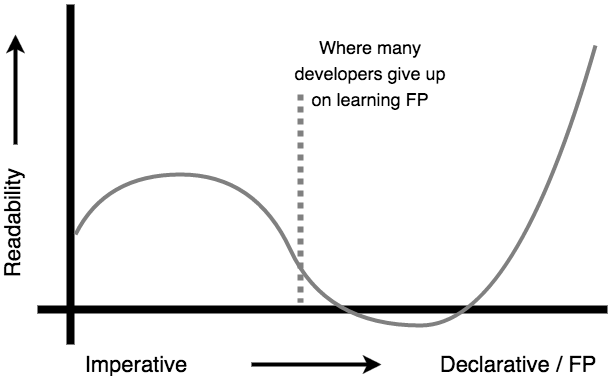### 函数式编程定义

JavaScript 是一门在设计之处就完全支持函数式编程的语言，在 JavaScript 里面，函数可以用 `function` 声明，作为全局变量，也就是这里说的“第一公民”。我们不再使用 `var``const` 或者 `let` 等关键字声明函数。我们也会大大减少变量的声明，通过函数的形参来替代变量的声明。

### 什么是函数？

``````y = f(x)
``````

``````function(x) { return y; }
``````

### 函数和程序的区别

• 程序是任意功能的合集，可以没有输入值，可以没有输出值。
• 函数必须有输入值和输出值。

### JavaScript 和函数式编程

JavaScript 支持函数式编程。使用 JavaScript 进行函数式编程时，我们要使用 JavaScript 的子集。不使用 for 循环, Math.random, Date, 不修改数据，来避免副作用，做到函数式编程。

### 高阶函数

``````[1, 2, 3].map(function(item, index, array) {
return item * 2;
});
``````
``````[1, 2, 3].reduce(function(accumulator, currentValue, currentIndex, array) {
return accumulator + currentValue;
}, 0);
``````

`map``reduce` 是高阶函数，它接收一个函数作为参数。

• 纯函数是幂等的
• 纯函数没有副作用

### 幂等

``````function add(a, b) {
return a + b;
}
``````

``````function add(a) {
return a + Math.random();
}
``````

``````var a = 1;
return a + b;
}
``````

``````var c = 1;
c++;
return a + b;
}
``````

• 纯函数是幂等的
• 纯函数没有副作用

### 使用不可变数据的准则

• 使用 `const`，不使用 `let`
• 不使用 `splice``pop``push``shift``unshift``reverse` 以及 `fill` 修改数组
• 不修改对象属性或方法

### 使用不可变数据的弊端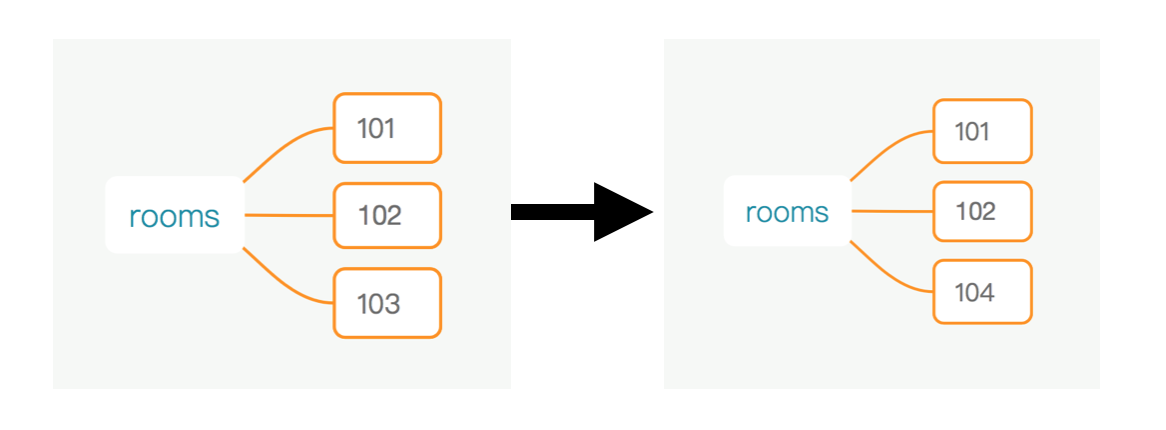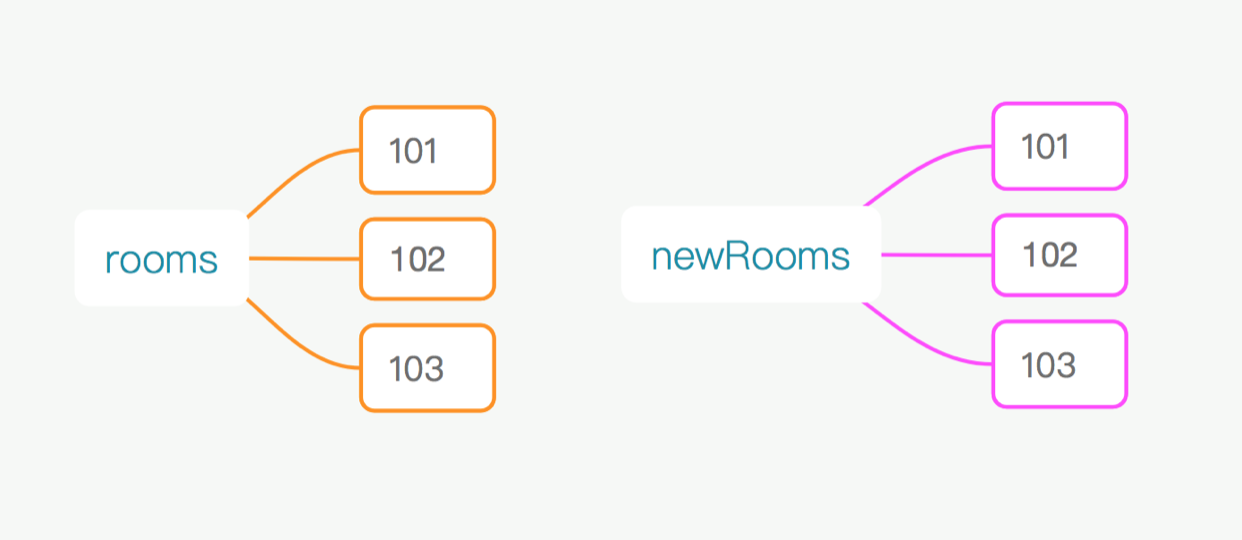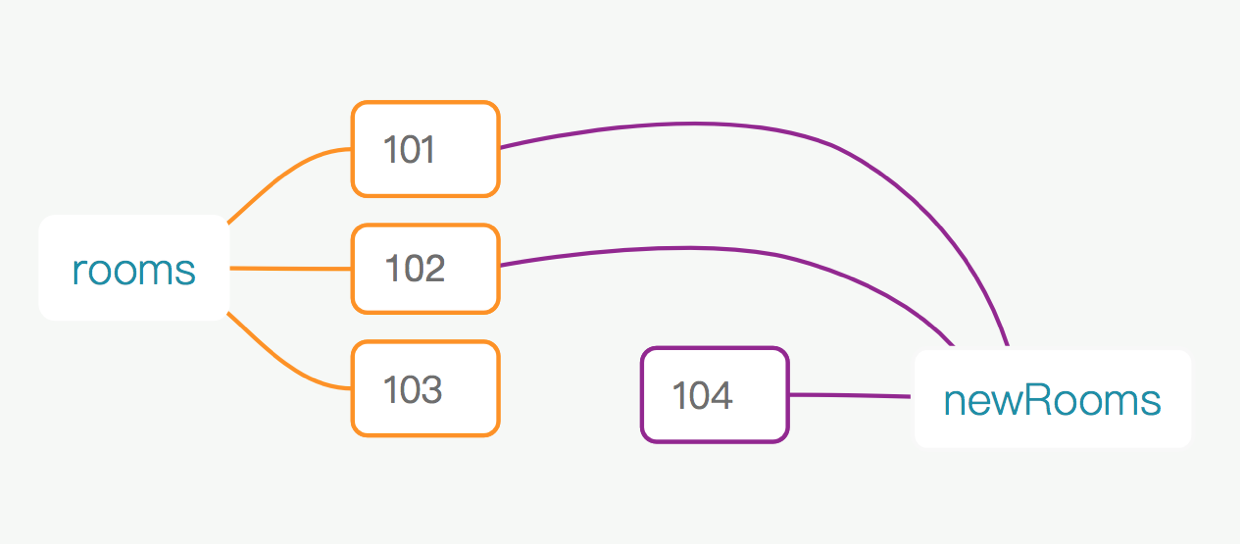### 闭包 vs 对象

``````var obj = {
one: 1,
two: 2
};

function run() {
return this.one + this.two;
}

var three = run.bind(obj);

three();		// => 3
``````
``````function getRun() {
var one = 1;
var two = 2;

return function run(){
return one + two;
};
}

var three = getRun();

three();			// => 3
``````

### 常见的辅助函数

• `unary`
• `reverseArgs`
• `curry`
• `uncurry`
• `compose`
• `pipe`
• `asyncPipe`

`unary``reverseArgs``curry``uncurry` 是用来进行参数操作的。`compose``pipe``asyncPipe` 是用来进行函数组合的。

#### `unary`

`unary` 是用来限制某个函数只接收一个参数的。常见的使用场景是处理 `parseInt` 函数：

``````['1', '2', '3'].map(parseInt);
// => [1, NaN, NaN]
``````
``````['1', '2', '3'].map(unary(parseInt));
// => [1, 2, 3]
``````

`unary` 的实现方式可以是：

``````const unary = (fn) => {
return (arg) => {
return fn(arg);
};
};
``````

#### `reverseArgs`

`reverseArgs` 是用来讲函数参数反转的，实现方式如下：

``````const reverseArgs = (fn) => {
return (...args) => {
return fn(...args.reverse());
};
};
``````

#### `curry`

`curry` 是用来把函数执行滞后的，让我们可以逐步把参数传入这个函数，当参数完整之后，目标函数才会执行。常见的用法如下：

``````function add(a, b) {
return a + b;
}
}
``````

``````function add(a, b) {
return a + b;
}
``````

`curry` 的实现思路如下：

`args`，保存起来，每个 `curried` 函数接受一个参数，将参数拼在之前的参数后面。

``````const curry = (fn) => {
const curried = (curArg) => {
const args = [...prevArgs, curArg];
return curried;
};
return curried;
};
``````

``````const curry = (fn) => {
return (curArg) => {
const args = [...prevArgs, curArg];
return nextCurried(...args);
};
};
``````

``````const curry = (fn) => {
const nextCurried = (...prevArgs) => {
return (curArg) => {
const args = [...prevArgs, curArg];
return nextCurried(...args);
};
};
return nextCurried();
};
``````

``````const curry = (fn, arity = fn.length) => {
const nextCurried = (...prevArgs) => {
return (curArgs) => {
const args = [...prevArgs, curArgs];
if (args.length >= arity) {
return fn(...args);
}
return nextCurried(...args);
};
};
return nextCurried();
};
``````

``````const curry = (fn, arity = fn.length) => {
const nextCurried = (...prevArgs) => {
return (...curArgs) => {
const args = [...prevArgs, ...curArgs];
if (args.length >= arity) {
return fn(...args);
}
return nextCurried(...args);
};
};
return nextCurried();
};
``````

#### `compose`

`compose` 用来串联执行函数，执行顺序是从后向前的。与之对应的是 `pipe` 函数，同样是串联执行函数，但是执行顺序是从前向后的。

`compose` 的用法：

``````function add10(value) {
return value + 10;
}
function multiple10(value) {
return value * 10;
}
``````

`compose` 的实现：

``````const compose = (...fns) => {
return fns.reduce((a, b) => {
return (...args) => {
return a(b(...args));
};
});
};
``````

``````const compose = (...fns) => {
return (input) => {
return fns.reduceRight((value, fn) => {
return fn(value);
}, input);
};
};
``````

#### `pipe`

`pipe` 也是用来组合函数的，串联执行的顺序是从前向后，与 `compose` 相反。`pipe` 的实现可以是：

``````const pipe = (...fns) => {
return fns.reduceRight((a, b) => {
return (...args) => {
return a(b(...args));
}
});
};
``````

`pipe` 的用法如下：

``````const addA = (value) => {
return value + 'A';
};
const addB = (value) => {
return value + 'B';
};
``````

#### `asyncPipe`

``````const asyncPipe =  (...fns) => {
return fns.reduceRight((next, fn) => {
return (...args) => {
fn(...args, next);
};
}, () => {});
};
``````

``````const addA = (value, next) => {
next(value + 'A', 'a');
};
const addB = (value, anotherValue, next) => {
console.log(anotherValue);                      // => a
next(value + 'B');
};
const consoleLog = (value, next) => {
console.log(value);
};

``````

### 函数式编程在数据结构上的运用

#### 实现链表

``````const createNode = (value, next) => {
return (x) => {
if (x) {
return value;
}
return next;
};
};
``````
``````const getValue = (node) => {
return node(true);
};
const getNext = (node) => {
return node(false);
};
``````
``````const append = (next, value) => {
if (next === null) {
return createNode(value, null);
}
return createNode(getValue(next), append(getNext(next), value));
};
const reverse = (linkedList) => {
return null;
}
};
``````
``````const linkedList1 = createNode(1, createNode(2, createNode(3, null)));
``````

### 参考资料

3 回复  |  直到 2019-10-29 10:41:40 +08:001 zppass   46 天前 牛批，看完后确实对函数式编程一些疑惑有了提升。2 DivineRapierH   46 天前 感谢大佬的分享！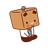3 564425833   42 天前 牛，我还以为最后有公众号。。